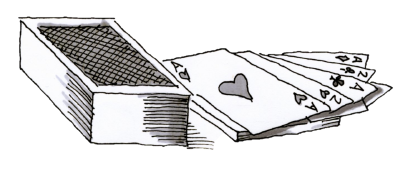### Home > CCAA > Chapter 5 Unit 3 > Lesson CC2: 5.2.1 > Problem5-30

5-30.

Imagine a standard deck of cards with all of the aces and twos removed. Find each probability below.

1. $\text{P(heart)}$

How many hearts are there (remember the ace and two have been removed)? What is the new total number of cards with the aces and twos removed?

$\frac{11}{44}$

2. $\text{P(black)}$

How many black cards are there (with aces and twos removed)? What is the new total number of cards now with the aces and twos removed?

3. $\text{P(face card)}$

What is the number of face cards? What is the new total number of cards?1. How is the $\text{P(face card)}$ different with this deck from the probability if the deck was not missing any cards? Which probability is greater? Why?

• What is the probability of drawing a face card when the deck is not missing any cards?

• Compare this probability with the one found in part (c).

• The probability of drawing a face card with aces and twos removed is higher because there are fewer non-face cards.

1. $\text{P(not heart)}$

What is $\text{P(heart)}$?
$\text{P(heart)}+ \text{P(not heart)}=1$

Since each suit loses the same number of cards, $\text{P(heart)}$ for this deck is the same as $\text{P(heart)}$ for a normal deck.